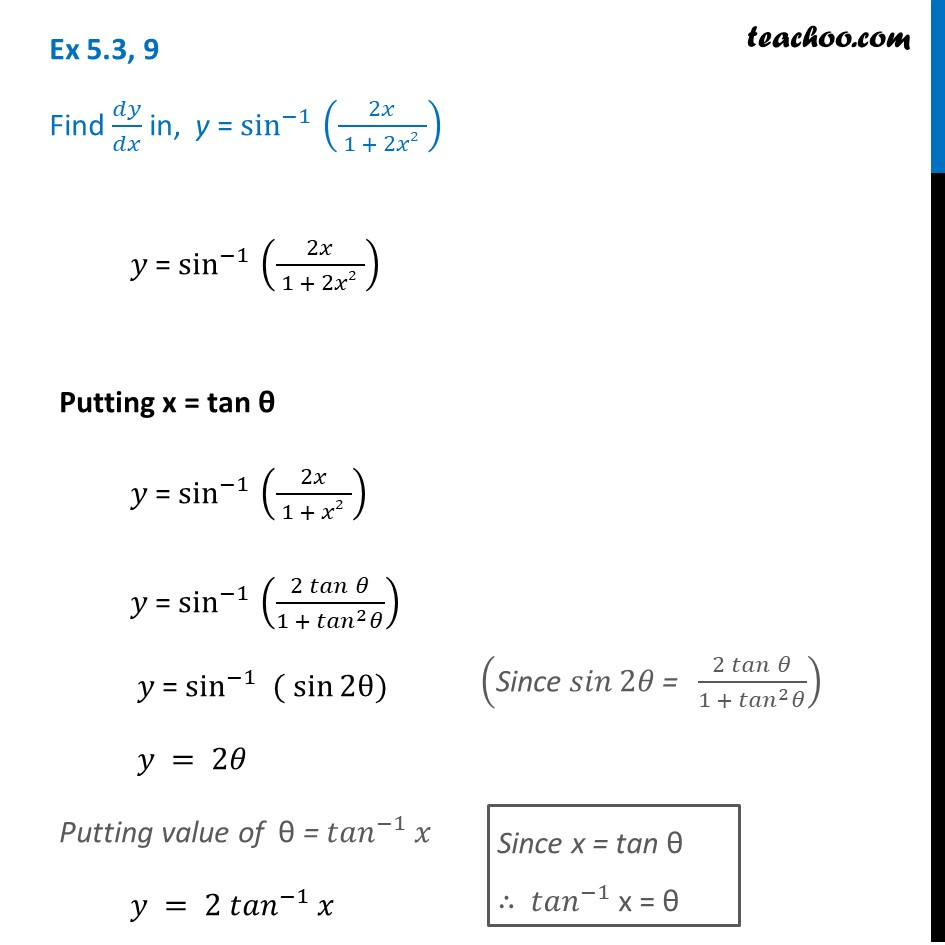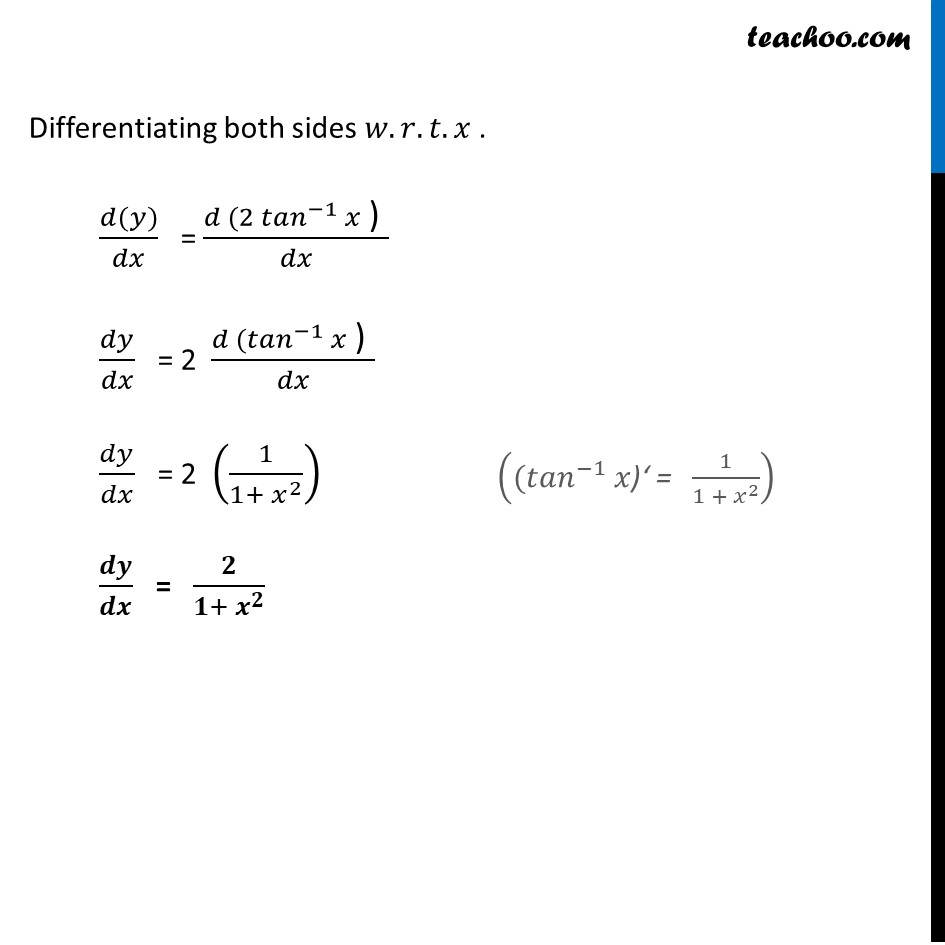Ex 5.3

Chapter 5 Class 12 Continuity and Differentiability
Serial order wiseLearn in your speed, with individual attention - Teachoo Maths 1-on-1 Class

### Transcript

Ex 5.3, 9 Find 𝑑𝑦/𝑑𝑥 in, y = sin^(−1) (2𝑥/( 1 + 2𝑥2 )) 𝑦 = sin^(−1) (2𝑥/( 1 + 2𝑥2 )) Putting x = tan θ 𝑦 = sin^(−1) (2𝑥/( 1 + 𝑥2 )) 𝑦 = sin^(−1) ((2 𝑡𝑎𝑛⁡𝜃)/(1 + 〖𝑡𝑎𝑛〗^2 𝜃)) 𝑦 = sin^(−1) ( sin⁡2θ) 𝑦 = 2𝜃 Putting value of θ = 〖𝑡𝑎𝑛〗^(−1) 𝑥 𝑦 = 2 〖𝑡𝑎𝑛〗^(−1) 𝑥 ("Since " 𝑠𝑖𝑛⁡2𝜃" = " (2 𝑡𝑎𝑛⁡𝜃)/(1 + 〖𝑡𝑎𝑛〗^2 𝜃)) Since x = tan θ ∴ 〖𝑡𝑎𝑛〗^(−1) x = θ Differentiating both sides 𝑤.𝑟.𝑡.𝑥 . (𝑑(𝑦))/𝑑𝑥 = (𝑑 (2 〖𝑡𝑎𝑛〗^(−1) 𝑥" ) " )/𝑑𝑥 𝑑𝑦/𝑑𝑥 = 2 (𝑑 (〖𝑡𝑎𝑛〗^(−1) 𝑥" ) " )/𝑑𝑥 𝑑𝑦/𝑑𝑥 = 2 (1/(1+ 𝑥^2 )) 𝒅𝒚/𝒅𝒙 = 𝟐/(𝟏+〖 𝒙〗^𝟐 ) ((〖𝑡𝑎𝑛〗^(−1) 𝑥")‘ = " 1/(1 + 𝑥^2 ))